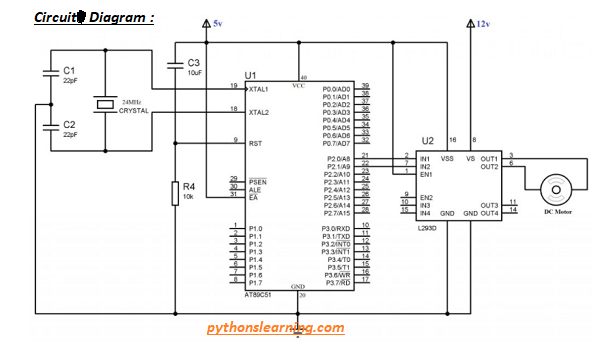How to 12 volt dc motor interfacing with 8051 using L293D

## How to 12 volt dc motor interfacing with 8051 using L293D

We know that in engineering life we used DC motor with 8051 microcontroller so For the beginner one question is Commonly ask How to connect DC motor to controller ?
or Can we directly connect DC motor to pic controller ? Answer is NO. because for 12 volt dc motor required 12v input supply it not drive directly on 15 mA at 5v

### How to drive DC motor with 8051 microcontroller

To remove or overcome this problem We can use L293D driver IC.
Explain L293D Driver IC
L293D Driver IC is a Quadruple Half H-Bridge driver and it solves the problem completely. You not need to connect any transistors, resistors or diodes. We can easily control the switching of L293D using a microcontroller.

### Block Diagram of dc motor interfacing with 8051 using L293D

Below block diagram or schematic show you how to connect dc motor to controller through L293D

As shown in figure EN1 is used to enable pair 1 (IN1-OUT1, IN2-OUT2) and EN2 is used to enable pair 2 (IN3-OUT3, IN4-OUT4). We can drive here only one DC motor. You can connect second DC Motor to driver pair 2 according to your needs.

### Source code for Drive 12 volt dc motor using L293D

``````#include<reg52.h>
#include<stdio.h>

void delay(void);

sbit motor_pin_1 = P2^0;
sbit motor_pin_2 = P2^1;

void main()
{
do
{
motor_pin_1 = 1;
motor_pin_2 = 0; //Rotates Motor Anit Clockwise
delay();
motor_pin_1 = 1;
motor_pin_2 = 1; //Stops Motor
delay();
motor_pin_1 = 0;
motor_pin_2 = 1; //Rotates Motor Clockwise
delay();
motor_pin_1 = 0;
motor_pin_2 = 0; //Stops Motor
delay();
}while(1);
}

void delay()
{
int i,j;
for(i=0;i<1000;i++)
{
for(j=0;j<1000;j++)
{
}
}
}``````

#### Compiler Output Windows :

Below windows shows that source code compile with zero error and Warning